# Financial Crisis Warning of Financial Robot Based on Artificial Intelligence

Financial Crisis Warning of Financial Robot Based on Artificial Intelligence
Accounting School, Wuhan College, Wuhan 430212, China

Corresponding Author Email:
9324@whxy.edu.cn
Page:
553-561
|
DOI:
https://doi.org/10.18280/ria.340504
17 May 2020
|
Accepted:
24 August 2020
|
Published:
20 November 2020
| Citation

OPEN ACCESS

Abstract:

Robotic process automation (RPA) financial robot provides a modern and intelligent tool for financial management, and financial business processing. Currently, more than 32% of financial applications are implemented by RPA financial robot. As an alternative of human in operation and judgment, the financial robot faces some inevitable risks in actual application. So far, there is a severe lack of theoretical or practical research into the risks or operational guarantees of RPA financial robot. To bridge the gap, this paper proposes a financial crisis warning model for financial robot based on artificial neural network (ANN), drawing on the merits of artificial intelligence (AI) like self-learning, self-adaptation, and self-adjustment. Specifically, a hierarchical evaluation index system (EIS) and the corresponding warning strategy were prepared for the financial crisis of RPA financial robot. Next, the financial crisis of RPA financial robot was evaluated both statically and dynamically. Then, antecedent and subsequent networks were merged into a fuzzy neural network (FNN) for predicting financial crisis of RPA financial robot. The proposed model was proved effective and accurate through experiments.

Keywords:

artificial intelligence (AI), financial robot, financial crisis warning, robotic process automation (RPA)

1. Introduction

Recent years has witnessed rapid development of big data analysis and cloud service systems. These advanced technologies have reshaped the form of financial sharing service, resulting in simpler business process, lower labor cost, and integrated financial information [1, 2]. Robotic process automation (RPA) financial robot provides a modern and intelligent tool for managing and processing financial businesses with large capacity, high repetition rate, and high error rate. Besides attracting wide academic attention, RPA financial robot has fundamentally promoted the digitalization and automation of traditional financial sharing services [3-5].

In June 2017, Deloitte launched its RPA financial robot. Many internationally renowned accounting firms have followed suit, including but not limited to PricewaterhouseCoopers, KPMG, and Ernst & Young. Their financial robots boast various pertinent functions and wide application scopes [6-9]. Currently, RPA financial robot is being adopted in more than 32% of financial applications [10-12]. However, the highly efficient financial robot brings some potential risks in dealing with extremely complex and important financial businesses, which demand a high processing accuracy [13-15]. It is of great necessity to realize the financial crisis warning of financial robot.

Many theoretical results have been achieved on financial crisis warning [16-18]. From the angle of cash flow, Lahmiri  analyzed the cash flow in each component of the total corporate value in business operations, and constructed a cash flow-based warning model for corporate financial risk. Cook and Watson  treated cash flow as the core index of financial crisis criteria, and mentioned several important impactors of financial crisis: the ratio of net cash flow to current liabilities, debt ratio, and return on investment (ROI). Using a five-variable model, De Pinho and Couto  discussed the possibility and influencing factors of financial crisis or bankruptcy in business operations, and divided the decisive factors into three aspects: registered capital and production scale, division of financial functions and management framework, as well as division of responsibilities and organizational structure.

On RPA financial decision-making, Sugiyanto et al.  studied the resource calling and management methods of two important parts of decision support system, namely, financial decision model library and database, and developed a reasonable functional architecture of human-machine conversation (HMC) following the conventional thinking of accounting business processing and management, highlighting that financial decision-making system cannot make the expected ideal decisions without the support from more advanced database technology. Kou et al.  suggested that an enterprise in financial crisis should simultaneously control internal financial risk and guard against external risk, and created a warning model for internal financial risk in structured operation. Andriosopoulos et al.  considered the mastery of advanced information technology (IT) the key to improve the internal risk control of enterprise group: the related financial risks between subsidiary and parent need to be controlled and handled through advanced IT, such that both sides in the enterprise group could operate stably. With the help of big data analysis and artificial intelligence (AI), Kanjilal and Ghosh  integrated the management and control mechanism of financial crisis into the state analysis of business management, assigned reasonable weights to warning indices through analytic hierarchy process (AHP), and realized the financial risk rating of actual operation and management states.

To sum up, the existing studies on RPA financial robot mainly focus on the opportunities and challenges brought by the robot to the accounting industry and accountants. There is a severe lack of theoretical or practical research into the risks or operational guarantees of RPA financial robot. To make up for the gap, this paper proposes an AI-based financial crisis warning model for financial robot, drawing on the merits of AI like self-learning, self-adaptation, and self-adjustment.

The remainder of this paper is organized as follows: Section 2 sets up a hierarchical evaluation index system (EIS) for the financial crisis of RPA financial robot, and puts forward the corresponding warning strategy; Section 3 carries out static and dynamic risk analyses for the financial crisis of RPA financial robot; Section 4 builds up a fuzzy neural network (FNN), which encompasses antecedent and subsequent networks, for predicting financial crisis of RPA financial robot; Section 5 verifies the effectiveness and accuracy of the proposed model; Section 6 puts forward the conclusions.

2. EIS Construction

Based on its application scenarios, the core functions of RPA financial robot can be divided into five dimensions: entry and query of financial data, processing and data recognition of texts and images, upload and download of system data stream, processing and analysis of financial data, and information monitoring and financial decision-making.

The function of entry and query of financial data replaces the manual operations of accountants to enter, migrate, and query financial data. The function of processing and data recognition of texts and images recognizes images and texts through optical character recognition (OCR), and obtains the valuable field information from texts and images, as well as the electronic financial data that can be directly structured. The function of upload and download of system data stream receives, uploads, downloads, and outputs financial data along the preset paths. The function of processing and analysis of financial data further screens, integrates, compares, verifies, and analyzes the output financial data. The function of information monitoring and financial decision-making promotes the progress of financial business with the aid of AI technology, or replace accountants to make reasonable financial decisions, while analyzing and monitoring financial data.

From the summary of the functions, it can be seen that RPA financial robot can bring convenience to businesses in several functional areas, including accounting department, human resources department, purchasing department, and customer service department. The technical operations of RPA financial robot fall into two modes: simulation of human operation and the simulation of human judgment. As an alternative of human in operation and judgment, the financial robot faces some inevitable risks in actual application.

Considering the application in various functional areas, the factors of RPA financial robot that might induce financial crisis were divided into eight categories: technical risk, application risk, control risk, business risk, compliance risk, organizational change risk, man-made risk, and sustainability risk. Among them, technical and application risks belong to system level; control and business risks belong to management level; the other risks belong to organization level. Our financial crisis warning strategy for RPA financial robot is illustrated in Figure 1.

## 1.png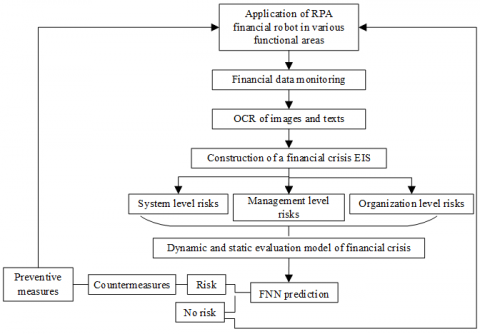Figure 1. The financial crisis warning strategy for RPA financial robot

Considering the features of the financial industry, the representativeness of risk elements, the sensitivity of crisis response, and availability of index data, the authors established a hierarchical EIS for financial crisis of RPA financial robot:

Layer 1 (goal): FC={crisis warning of financial robot}

Layer 2 (primary indices):

FC={FC1, FC2, FC3, FC4, FC5, FC6, FC7, FC8}={technical risk, application risk, control risk, business risk, compliance risk, organizational change risk, man-made risk, sustainability risk};

Layer 3 (secondary indices):

FC1={FC11, FC12, FC13, FC14, FC15, FC16, FC17, FC18, FC19}={technical maturity, functional availability, system failure rate, maintenance mechanism, crisis response plan, resource allocation rationality, business capabilities, user permissions, data transmission security};

FC2={FC21, FC22, FC23}={application rationality, application process stability, ROI};

FC3={FC31, FC32, FC33}={application automation, design and programing risk, control system rationality};

FC4={FC41, FC42}={information process interruption, emergency program};

FC5={FC51, FC52, FC53, FC54, FC55, FC56}={application process rationality, operation interference, system error, using permissions, data logic rule rationality, financial standardization scheme rationality};

FC6={FC61, FC62, FC63}={ post change, strategic adjustment, career growth};

FC7={FC71, FC72, FC73, FC74}={business process proficiency, operational logic understanding, operation familiarity, operation error rate};

FC8={FC81, FC82, FC83, FC84}={power supply, resource supply, emphasis on application, planning and promotion strategy}.

The above EIS contains 8 primary indices and 34 secondary indices. Thus, the financial crisis warning is a multi-parameter comprehensive evaluation problem. However, the evaluation indices differ in attribute and dimension. The original data of these indices must be normalized to reflect the financial risks in actual scenarios.

Firstly, an original evaluation matrix FC=[FCij]m×n was constructed according to the EIS. Then, the fitness indices like system failure rate, ROI, and business proficiency in the EIS were processed by max-min strategy (MMS):

$\begin{equation} F C^{*}=\frac{1}{\left|F C_{i j}-F C_{o p t}\right|} \end{equation}$     (1)

where, FC* is the value of a fitness index after MMS processing; FCopt is the industry standard value (theoretical optimal value) of primary index FCj. After MMS processing, the index data were nondimensionalized by extreme value method:

$\begin{equation} F C_{i j}^{*}=\frac{F C_{i j}-F C_{\max , j}}{F C_{\max , j}-F C_{\min . j}} \end{equation}$     (2)

where, FC*ij is a value in the interval [0, 1]; FCij is the original data of the evaluation index; FCmax-j=max{FCj} and FCmin-j=min{FCj} are the maximum and minimum assigned by experts to the primary index FCj for the financial crisis of financial robot, respectively. Without loss of generality, the normalized data of the evaluation index is still expressed as FCij.

3. Dynamic and Static Evaluations

3.1 Static evaluation

During the dynamic financial crisis evaluation of RPA financial robot, the evaluation indices involve many time series data (Table 1).

Table 1. The time series data for financial crisis evaluation of RPA financial robot

 Time Period 1 Period 2 … Period P FC1 FC111, FC112, …, FC11M FC211, FC212, …, FC21M … FCP11, FCP12, …, FCP1M FC2 FC121, FC122, …, FC12M FC221, FC222, …, FC22M … FCP21, FCP22, …, FCP2M ⋮ ⋮ ⋮ … ⋮ FCN FC1N1, FC1N2, …, FC1NM FC2N1, FC2N2, …, FC2NM … FCPN1, FCPN2, …, FCPNM

Let FC={FCk|k=1,2,…,N} be the set of primary indices, FCk={FCkl|k=1,2,…,N;l=1,2,…,M} be the set of secondary indices, and ET={t|t=1,2,…,P} be the set of evaluation periods, where FCtkl is the original data on secondary index FCkl at time t. Then, the static evaluation matrix for financial crisis of RPA financial robot at time t can be expressed as:

$\begin{equation} F C^{t}=\left[F C_{k l}^{t}\right]_{N \times M}=\left[\begin{array}{cccc} F C_{11}^{t} & F \mathrm{C}_{12}^{t} & \cdots & F \mathrm{C}_{1 M}^{t} \\ F C_{21}^{t} & F \mathrm{C}_{22}^{t} & \cdots & F \mathrm{C}_{2 M} \\ \vdots & \vdots & \cdots & \vdots \\ F C_{N 1}^{t} & F \mathrm{C}_{N 2}^{t} & \cdots & F \mathrm{C}_{N M}^{t} \end{array}\right] \end{equation}$     (3)

Suppose the set of secondary indices FCk contains M nodes (secondary indices) FCkl. Then, the connection relationship between nodes FCkx and FCky (x,y=1, 2, …, M) can be expressed as:

$\begin{equation} C R_{x y}=\left\{\begin{array}{ll} 1 & \text { Connect } \\ 0 & \text { No connect } \end{array}\right. \end{equation}$     (4)

The correlation coefficient between index vectors FCka and FCkb can be computed by:

$\begin{equation} \mu_{x y}^{t}=\frac{\sum_{i=1}^{n}\left(F C_{i x}^{t}-F C_{x}^{t}\right)\left(F C_{i y}^{t}-F C_{y}^{\prime t}\right)}{\sqrt{\sum_{i=1}^{n}\left(F C_{i x}^{t}-F C_{x}^{\prime t}\right)^{2} \cdot \sum_{i=1}^{n}\left(F C_{i y}^{t}-F C_{y}^{\prime t}\right)^{2}}} \end{equation}$     (5)

where,

$\begin{equation} \left\{\begin{array}{l} F C_{x}^{\prime t}=\frac{1}{N} \sum_{i=1}^{N} F C_{i x}^{t} \\ F C_{y}^{\prime t}=\frac{1}{N} \sum_{i=1}^{N} F C_{i y}^{t} \end{array}\right. \end{equation}$     (6)

Then, the correlation coefficient μtxy was normalized with zero as the limit. If μtxy>0, nodes FCkx and FCky are connected, and CRtxy and CRtyx are both one; if μtxy<0, nodes FCkx and FCky are not connected, and CRtxy and CRtyx are both zero. On this basis, a symmetric correlation matrix CRt can be established as:

$\begin{equation} C R^{t}=\left[C R_{x y}^{t}\right]_{M \times M}=\left[\begin{array}{rccc} / & C R_{12}^{t} & \cdots & C R_{1 M}^{t} \\ C R_{21}^{t} & / & \cdots & C R_{2 M}^{t} \\ \vdots & \vdots & \vdots & \vdots \\ C R_{M 1}^{t} & C R_{M 1}^{t} & \cdots & / \end{array}\right] \end{equation}$     (7)

For the node set FCk={FCkl|k=1, 2, …, N; l=1,2,…,M}, the centrality CENtkl of each node can be calculated by:

$\begin{equation} C E N_{j}^{t}=\frac{\sum_{x \neq y, y=1}^{M} C R_{x y}^{t}}{M-1} \end{equation}$     (8)

The normalized centrality of node FCkl is the weight of that node at time t:

$\begin{equation} \omega_{k l}^{t}=C E N_{k l}^{t} / \sum_{l=1}^{M} C E N_{k l}^{t} \end{equation}$     (9)

where, ωtkl is within the interval (0, 1). At time t, the weights of secondary indices for financial crisis of RPA financial robot can be expressed as ωtk=(ωtk1, ωtk2, …, ωtkM).

To improve the evaluation accuracy and simplify the processing of index data, the closely correlated indices were allocated to multiple clusters, and the size and mass features of each cluster were fused; after that, the density operator was adopted to measure the sparsity of financial crisis information in each cluster. The density weight ρtg of mass feature of cluster FCk={FCkl|k=1, 2, …, N; l=1,2,…,Q} can be calculated by:

$\begin{equation} \rho_{g}^{t}=C O H_{g}^{t} / \sum_{g=1}^{Q} C O H_{g}^{t} \end{equation}$     (10)

where, Q is the number of nodes in cluster FCk; ρtg∈(0, 1) is the density weight of mass feature; COHtg is the cohesion of cluster FCg at time t, reflecting the density of the nodes in the cluster. The higher the COHtg value, the more consistent the financial crisis information in the evaluation indices, and the better the clustering effect. The COHtg value can be calculated by:

$\begin{equation} C O H_{g}^{t}=\frac{\sum_{x \in R} \sum_{y \in R} C R_{x y}^{t}}{R(R-1)} \end{equation}$     (11)

where, R is the number of nodes in cluster FCg; CRtxy is the element value obtained through multiple iterations of the correlation matrix. Let θtg=(Ru/M)B be the weight impact factor. The density weight φtg of the size feature of cluster FCk={FCkl|k=1,2, …, N; l=1,2,…,Q} can be calculated by:

$\begin{equation} \phi_{g}^{t}=\frac{\theta_{g}^{t}\left(R_{g} / M\right)}{\sum_{g=1}^{Q} \theta_{g}^{t}\left(R_{g} / M\right)} \end{equation}$     (12)

where, B is the density impact index. Let υtg={υ1t,υ2t…,υtp} be the weighted vector of index density in each cluster. Then, the cohesion of cluster FCg can be obtained by aggregating the density weights of mass feature and size feature through multiplicative normalization:

$\begin{equation} v_{g}^{t}=\frac{\rho_{g}^{t} \phi_{g}^{t}}{\sum_{u=1}^{Q} \rho_{g}^{t} \phi_{g}^{t}} \end{equation}$     (13)

Then, the static evaluation value of financial crisis of RPA financial robot at each moment can be calculated by:

$\begin{equation} E_{k}^{t}=\sum_{g=1}^{Q} v_{g}^{t}\left(\sum_{k=1}^{R} \omega_{k}^{g t} F C_{k l}^{t}\right) \end{equation}$     (14)

where, Etk is the static evaluation value of primary index FCk (the greater the value, the more unlikely the financial crisis); ωgtk is the weight of normalized index FCtkg in cluster FCtg.

3.2 Dynamic evaluation

Based on the static evaluation, the dynamic evaluation for financial crisis of RPA financial robot comprehensively considers the timeliness of each index and the volatility of each functional area (Table 2). The time weight of each index can be obtained ideally by:

$\begin{equation} \max E N T=-\sum_{i=1}^{T} \tau_{t} \ln \tau_{t} \end{equation}$     (15)

where, ENT is the entropy of time vector that satisfies:

$\begin{equation} \left\{\begin{array}{l} \alpha=\frac{1}{T-1} \sum_{t=1}^{T}(T-t) \tau_{t}, 0 \leq \alpha \leq 1 \\ \tau_{a}-\tau_{b}>0,(a, b \in T, a>b) \\ \sum_{t=1}^{T} \tau_{t}=1, \tau_{t} \in[0,1] \end{array}\right. \end{equation}$     (16)

where, α is the degree of time (the closer α is to 1, the more important the earlier information of the index); ζt is the time weight reflecting the volatility of each functional area. The ζt value can be determined based on the information entropy of the static evaluation value:

$\begin{equation} \zeta_{t}=1-E N T_{t} \end{equation}$     (17)

where, ENTt is the entropy of time vector at time t. The smaller the ζt value, the smaller the gap of Etk, the less volatile each functional area.

Table 2. The classification criteria for the trends of financial crisis

 Type Criterion Meaning Rising risk Degree of time < 0.5; entropy>0.5 Financial crisis tends to worsen, and dynamic risk grows with time. Falling risk Degree of time> 0.5; entropy<0.5 Financial crisis tends to improve, and dynamic risk falls with time. Volatile environment Entropy $\begin{equation} \in \end{equation}$ (0.5, 1) The functional areas are highly volatile, and financial risk exhibits an uncertain trend. Time insensitivity Degree of time $\begin{equation} \in \end{equation}$ (0.5, 1) The trend of financial crisis is uncertain and insensitive to time.

After integrating the timeliness of each index and the volatility of each functional area, the time weight can be expressed as:

$\begin{equation} \psi_{t}=a \cdot \tau_{t}+b \cdot \zeta_{t} \end{equation}$     (18)

where, a and b are both numbers in (0, 1); a + b = 1. The dynamic evaluation value of financial crisis for RPA financial robot can be calculated by:

$\begin{equation} D E_{k l}=\sum_{t=1}^{T} \psi_{t} y_{k l}^{t} \end{equation}$     (19)

DEkl characterizes the dynamic risk of financial crisis. The greater the DEkl value, the smaller the risk; the smaller the DEkl value, the greater the probability of financial crisis.

4. Prediction Model

The FNN integrates the merits of fuzzy theory and artificial neural network (ANN). Besides its excellence in self-learning and association, the FNN makes full use of expert knowledge through a simple reasoning process, without strict requirements on samples. This paper combines the antecedent and subsequent networks into an FNN (Figure 2).

The input layer receives the dynamic evaluation values of the indices for the financial crisis of RPA financial robot. These values can be expressed as I=[I1,I2,…,IN]. In the input layer, N1 nodes process the input data, and transfer the results to the fuzzification layer. The membership function between each input data and its corresponding linguistic fuzzy set can be described as a Gaussian function:

$\begin{equation} \mu_{i}^{j}=e^{-\left(I_{i}-P_{i j}^{2}\right) / \varepsilon_{i j}} \end{equation}$     (20)

where, εij is the width of the Gaussian membership function; Pij is the x-coordinate of the center of the function; i=1, 2, …, N; j=1, 2, …, M. Note that N is the dimension of the input data; M is the number of fuzzy classes. Table 3 lists the fuzzy classes and risk levels of financial crisis of RPA financial robot.

## 2.png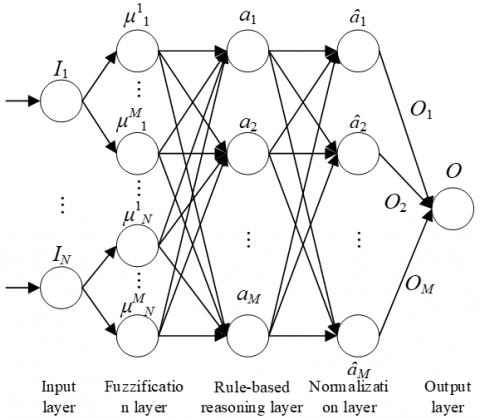Figure 2. The structure of the proposed FNN

Table 3. The fuzzy classes and risk levels

 Fuzzy class Risk level Meaning 1 E Strongly low system risk, strongly low management risk, and strongly low organization risk 2 D Slightly low system risk, slightly low management risk, and slightly low organization risk 3 C Neutral system risk, neutral management risk, neutral organization risk 4 B Slightly high system risk, slightly high management risk, and slightly high organization risk 5 A Strongly high system risk, strongly high management risk, and strongly high organization risk

In the fuzzification layer, N2 nodes process the received data, and push the results to the rule-based reasoning layer. In the rule-based reasoning layer, the N3=M nodes correspond to the fuzzy rules for the dynamic evaluation of the financial crisis, and compute the fitness of each fuzzy rule:

$\begin{equation} a_{j}=\mu_{1}^{r_{1}} \cdot \mu_{2}^{r_{2}} \cdots \mu_{N}^{r_{N}} \end{equation}$     (21)

where, r1Î{1,2,…,Q1}, rN∈{1,2,…,QN}, j=1, 2, …, M. The number of nodes in the rule-based reasoning layer equals the continuous multiplication of Q1 to QN. The results from the rule-based reasoning layer are forwarded to the normalization layer, where N4=N3=M nodes normalizes the received data:

$\begin{equation} \hat{a}_{i}=\alpha_{j} / \sum_{i=1}^{M} a_{i} \end{equation}$     (22)

The normalized results are defuzzied and outputted by the output layer. The output can be computed by:

$\begin{equation} O=\sum_{i=1}^{M} O_{i} \hat{a}_{i} \end{equation}$     (23)

The FNN was designed in reference to the structure of backpropagation neural network (BPNN). The error objective function of the FNN can be expressed as:

$\begin{equation} E=\frac{1}{2}\left(O^{*}-O\right)^{2} \end{equation}$     (24)

where, O* and O are the expected output and actual output of the FNN, respectively. Following the idea of BPNN algorithm, the connection weights ωji of the output layer, the width εij of the membership function, and the center Pij of the membership function were adjusted. The learning algorithm of ωji can be expressed as:

$\begin{equation} \frac{\partial E}{\partial \omega_{j i}}=\frac{\partial E \partial O \partial O_{j}}{\partial O \partial O_{j} \partial O_{j i}}=-\left(O^{*}-O\right) \hat{a}_{j} I_{i} \end{equation}$     (25)

$\begin{equation} \omega_{j i}(k+1)=\omega_{j i}(k)-\eta \frac{\partial E}{\partial \omega_{j i}} \end{equation}$     (26)

where, η is the learning rate. Fixing the weight ωji, εij and Pij can be respectively adjusted by:

$\begin{equation} \varepsilon_{i j}(k+1)=\varepsilon_{i j}(k)-\eta \frac{\partial E}{\partial \varepsilon_{i j}} \end{equation}$     (27)

$\begin{equation} P_{i j}(k+1)=P_{i j}(k)-\eta \frac{\partial E}{\partial P_{i j}} \end{equation}$     (28)

5. Experiments and Results Analysis

## 3.png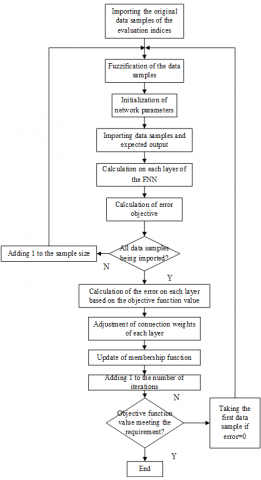Figure 3. The training flow of the FNN

As shown in Figure 3, the BPNN-based FNN was trained in the following process. First, the data samples on the evaluation indices for the financial crisis of RPA financial robot are fuzzified. Then, the network parameters are initialized, and the data samples are imported, together with the expected output. After that, each layer of the FNN performs calculations, and derives the value of the objective function. After the network is trained by all data samples, the output error of each layer is computed according to the objective function value. Then, the connection weights of each layer are adjusted, followed by the update of the membership function. Through repeated iterations, the objective function value eventually reaches the preset requirement.

The training and test errors of our model are shown in Figures 4(a) and 4(b), respectively, where x-axis is the training cycle, and y-axis is the RMSE. The data samples on the evaluation indies for the financial crisis of RPA financial robot in an enterprise were split into a training set and a test set at the ratio of 4:1. As shown in Figure 4, the training effect was satisfactory after 7-8 iterations in training or testing. The trained network achieved good approximation accuracy, indicating that our model can effectively fit the training and test samples. Figure 5 displays the convergence effect of our model. It can be seen that the training error tended to be stable, after the FNN iterated for about 100-140 times.

Figure 6 shows how the node centrality of the dynamic evaluation model affects the training error of the FNN. With the growing node centrality, the training error gradually decreased. Thus, the proposed model can optimize node centrality to a certain extent.

## 4-1.png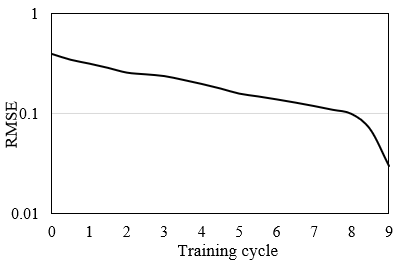(a) Training error

## 4-2.png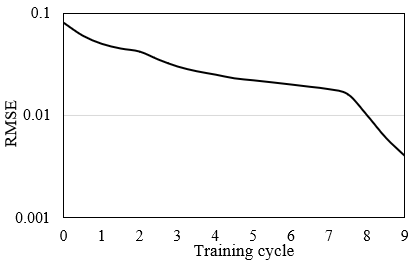(b) Test error

Figure 4. The training error and test error of our model

Note: RMSE is root mean square error

Figure 7 presents the risk prediction results of our model on some samples. It can be seen that the predicted value on test data exhibited a similar trend as that on training data. This means the proposed model has a high test accuracy; the pretrained FNN can ideally approximate the actual values of the data samples on evaluation indies.

## 5.pngFigure 5. The convergence effect of our model

## 6.png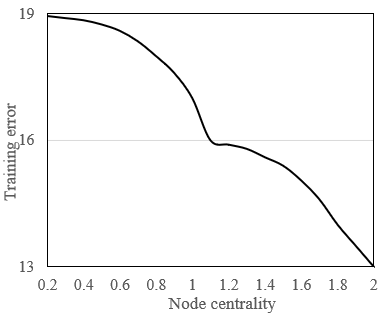Figure 6. The influence of node centrality on training error

## 7.png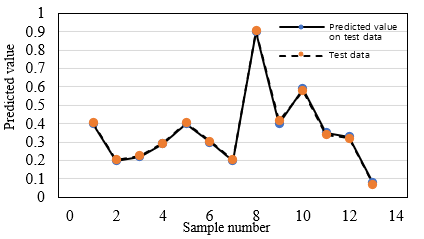Figure 7. The risk prediction of our model (part)

Figures 8(a) and 8(b) provide the fuzzy classification results of our model on training and test data, respectively. It can be seen that deviations appeared on 4 samples in the training set, and 4 samples in the test set. But the deviations were controlled near the value range of the correct class. The high consistency verifies the recognition ability, credibility, and feasibility of the proposed model.

## 8-1.jpg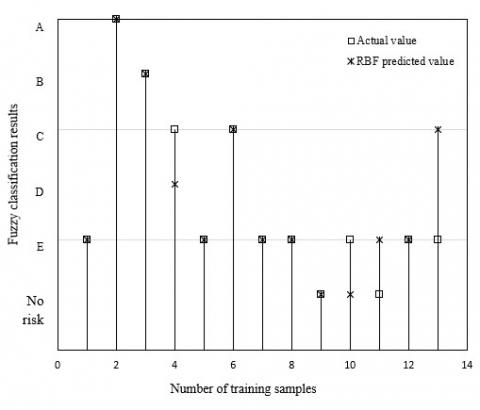(a) Training data

## 8-2.jpg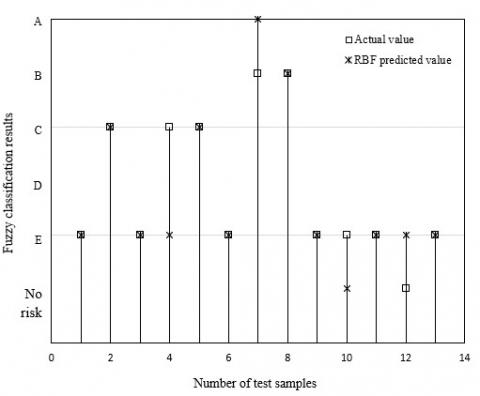(b) Test data

Figure 8. The fuzzy classification results of our model on training and test data

The dynamic evaluation results in 2019 were sorted out by month (Table 4). It can be seen that the risk of financial crisis was high in January, February, August, and October. Compared with the static evaluation results, the dynamic evaluation results are close to the reality. Therefore, it is scientific for the dynamic evaluation model to consider the considers the timeliness of each index and the volatility of each functional area.

For comparison, our model was compared with several traditional models in accuracy, recall, F-score, mean absolute error (MAE), and RMSE: BPNN + static evaluation, long short-term memory (LSTM) + static evaluation, FNN + static evaluation, and BPNN + dynamic evaluation. The evaluation results of these models are compared in Table 5.

As shown in Table 5, BPNN + dynamic evaluation and our model both surpassed 0.85 in accuracy, recall, and F-score, reflecting the accuracy and feasibility of the dynamic evaluation model for financial crisis of RPA financial robot. In addition, our model outperformed the BPNN + dynamic evaluation, as evidenced by its relatively low error and high accuracy.

Table 4. The dynamic evaluation results in 2019

 Month Level Meaning 2019-01 A High risk 2019-02 A High risk 2019-03 B Moderate risk 2019-04 C General risk 2019-05 D Low risk 2019-06 C General risk 2019-07 D Low risk 2019-08 A High risk 2019-09 B Moderate risk 2019-10 A High risk 2019-11 B Moderate risk 2019-12 C General risk

Table 5. The evaluation results of different models

 Accuracy Recall F-score MAE RMSE BPNN + static evaluation 0.765 0.801 0.747 0.228 0.234 LSTM + static evaluation 0.798 0.811 0.791 0.105 0.142 FNN + static evaluation 0.803 0.823 0.825 0.121 0.126 BPNN + dynamic evaluation 0.855 0.861 0.852 0.095 0.103 Our model 0.892 0.881 0.869 0.012 0.016
6. Conclusions

In data processing, the AI has such advantages as self-learning, self-adaptation, and self-adjustment. Therefore, this paper establishes a financial crisis warning model for financial robot based on AI. Firstly, a scientific hierarchical EIS was created for the financial crisis of RPA financial robot, and the corresponding warning strategy was provided. On this basis, the financial crisis of RPA financial robot was evaluated statically and dynamically. Then, antecedent and subsequent networks were combined into an FNN for predicting the financial crisis of RPA financial robot. Experimental results demonstrate that the proposed model can desirably fit the training and test samples, and outperform the other models in error and accuracy.

References

 Cardenas, I.S., Kim, J.H. (2020). Robonomics: The study of robot-human peer-to-peer financial transactions and agreements. ACM/IEEE International Conference on Human-Robot Interaction, pp. 8-15.

 Bataev, A.V., Dedyukhina, N., Nasrutdinov, M.N. (2020). Innovations in the financial sphere: Performance evaluation of introducing service robots with artificial intelligence. ICITM 2020 - 2020 9th International Conference on Industrial Technology and Management, IEEE, Oxford, United Kingdom, pp. 256-260. https://doi.org/10.1109/ICITM48982.2020.9080379

 Cartlidge, J., Cliff, D. (2013). Evidencing the "robot phase transition" in human-agent experimental financial markets. ICAART 2013 - Proceedings of the 5th International Conference on Agents and Artificial Intelligence, 1: 345-352.

 De Luca, M. (2015). Why robots failed: Demonstrating the superiority of multiple-order trading agents in experimental human-agent financial markets. ICAART 2015 - 7th International Conference on Agents and Artificial Intelligence, Proceedings, 1: 44-53.

 Malyarenko, A., Nohrouzian, H., Silvestrov, S. (2020). An algebraic method for pricing financial instruments on post-crisis market. Springer Proceedings in Mathematics and Statistics, 317: 839-856. https://doi.org/10.1007/978-3-030-41850-2_35

 Azadian, F. (2020). Assessing the effect of urban socioeconomic factors and the financial crisis of 2008 on domestic air cargo traffic in Florida. Transportation, 47(1): 223-241. https://doi.org/10.1007/s11116-018-9872-9

 Upadhyay, S., Banerjee, A., Panigrahi, P.K. (2020). Causal evolution of global crisis in financial networks. Physica A: Statistical Mechanics and its Applications, 554: 124690. https://doi.org/10.1016/j.physa.2020.124690

 Wang, G., Wang, K.M., Zhou, Y.Y., Mo, X.Y. (2020). Establishment of a financial crisis early warning system for domestic listed companies based on three decision tree models. Mathematical Problems in Engineering. https://doi.org/10.1155/2020/8036154

 Uthayakumar, J., Metawa, N., Shankar, K., Lakshmanaprabu, S.K. (2020). Financial crisis prediction model using ant colony optimization. International Journal of Information Management, 50: 538-556. https://doi.org/10.1016/j.ijinfomgt.2018.12.001

 Joo, K., Suh, J.H., Lee, D.Y., Ahn, K. (2020). Impact of the global financial crisis on the crude oil market. Energy Strategy Reviews, 30: 100516. https://doi.org/10.1016/j.esr.2020.100516

 Liu, H.Y., Song, Y.R. (2020). Financial development and carbon emissions in China since the recent world financial crisis: Evidence from a spatial-temporal analysis and a spatial Durbin model. Science of the Total Environment, 715: 136771. https://doi.org/10.1016/j.scitotenv.2020.136771

 Sankhwar, S., Gupta, D., Ramya, K.C., Rani, S.B., Shankar, K., Lakshmanaprabu, S.K. (2020). Improved grey wolf optimization-based feature subset selection with fuzzy neural classifier for financial crisis prediction. Soft Computing, 24(1): 101-110. https://doi.org/10.1007/s00500-019-04323-6

 Andreoni, V. (2020). The energy metabolism of countries: Energy efficiency and use in the period that followed the global financial crisis. Energy Policy, 139: 111304. https://doi.org/10.1016/j.enpol.2020.111304

 Majapa, M., Gossel, S. (2016). Topology of the South African stock market network across the 2008 financial crisis. Physica A: Statistical Mechanics and its Applications, 445: 35-47. https://doi.org/10.1016/j.physa.2015.10.108

 Da Silva, M.F., Pereira, E.J.D.A.L., Filho, A.M.D.S., Castro, A.P.N., Miranda, J.G.V., Zebende, G.F. (2016). Quantifying the contagion effect of the 2008 financial crisis between the G7 countries. Physica A: Statistical Mechanics and its Applications, 453: 1-8. https://doi.org/10.1016/j.physa.2016.01.099

 Kuppuswamy, V., Villalonga, B. (2016). Does diversification create value in the presence of external financing constraints? Evidence from the 2007-2009 financial crisis. Management Science, 62(4): 905-923. https://doi.org/10.1287/mnsc.2015.2165

 Ma, P.C., Li, D.Y., Li, S. (2016). Efficiency and cross-correlation in equity market during global financial crisis: Evidence from China. Physica A: Statistical Mechanics and its Applications, 444: 163-176. https://doi.org/10.1016/j.physa.2015.10.019

 Park, S.J., Cho, K.H. (2016). Power-based supervisory control theory of hybrid systems and its application to the analysis of financial crisis. IET Control Theory and Applications, 10(7): 780-788. https://doi.org/10.1049/iet-cta.2015.1047

 Lahmiri, S. (2017). A study on chaos in crude oil markets before and after 2008 international financial crisis. Physica A: Statistical Mechanics and its Applications, 466: 389-395. https://doi.org/10.1016/j.physa.2016.09.031

 Cook, S., Watson, D. (2017). Revisiting the returns–volume relationship: Time variation, alternative measures and the financial crisis. Physica A: Statistical Mechanics and its Applications, 470: 228-235.

 De Pinho, F.M., Couto, R.F. (2017). Comparing volatility forecasting models during the global financial crisis. Communications in Statistics: Simulation and Computation, 46(7): 5257-5270. https://doi.org/10.1080/03610918.2016.1152363

 Sugiyanto, Zukhronah, E., Susanti, Y., Dwi, S.R. (2017). Markov switching autoregressive conditional heteroscedasticity (SWARCH) model to detect financial crisis in Indonesia based on import and export indicators. Journal of Physics: Conference Series, 855.

 Kou, S., Yu, C., Zhong, H.W. (2017). Jumps in equity index returns before and during the recent financial crisis: A Bayesian analysis. Management Science, 63(4): 988-1010. https://doi.org/10.1287/mnsc.2015.2359

 Andriosopoulos, K., Galariotis, E., Spyrou, S. (2017). Contagion, volatility persistence and volatility spill-overs: The case of energy markets during the European financial crisis. Energy Economics, 66: 217-227. https://doi.org/10.1016/j.eneco.2017.06.023

 Kanjilal, K., Ghosh, S. (2017). Dynamics of crude oil and gold price post 2008 global financial crisis – New evidence from threshold vector error-correction model. Resources Policy, 52: 358-365. https://doi.org/10.1016/j.resourpol.2017.04.001